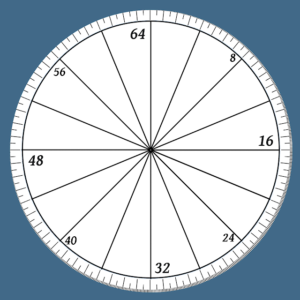Categories

# Compass bearings as 64 parts.

As is the custom, the Friday blog will be a little more random and off-the wall than usual. Some time back I had some fun creating a system of weights and measures for a fictional environment. I based this system on the human pace and cubit length and derived a related system of mass and volume measurement.

The other night I was lying awake in bed and began to wonder about other alternate systems of measure. Specifically I began to think about directional bearings.

The system most familiar to many of us is that of degrees, there being 360 degrees in a circle. One theory is that there are 360 degrees in a circle because the sun appears to move approximately one degree each day. Another theory is that six equilateral triangles will make up a full circle. Each segment was then further divided into 60 by the Babylonians, who liked to do things in 12s and 60s. Incidentally the Russians also had a system based on six triangles but divided each into 100 to give 600 increments in a circle.

There didn’t seem to be any particular reason why a culture would select a system based on 360, 36, 60 or 12. Generally we think of a circle having four cardinal directions and a circle is easily divided into quarters. Each quarter is one right angle. The quarters are easily bisected to give us eight equal segments of a circle, an eighth being half a right angle. The eighths can be bisected again to give us 16 parts. If we divide the rim of each sixteenth into four equal parts we have divided the circle into 64.
I have not been able to find a good word to denote a sixty-forth part. Closest thing I came across was a “semifusa”, itself a fraction of larger measurement. For want of a better term I will call a 64th of a circle’s circumference a “Rey”.
Breaking the circle up into 64 gives us quite an elegant system. A right angle or quarter circle is 16/64ths or 16 Rey. Half a right angle or an eighth of a circle is 8 Rey. Many other useful increments are also nice whole numbers. Bearings are given in Rey. “Quarter” is a permissible alternate term for right angles, “eighth” for half right angles. Smaller angles such as sixteenths or thirty-seconds are instead given in multiples of Rey. One Rey is 5.625 degrees, a nice sized unit to work with.The Rey or 64th can of course be divided further for more accuracy. Rather than continuing the process of further halving the unit my inclination was to decimalize fractions of the Rey. Bearings could be expressed as 9.3 Rey, for example, each tenth of a Rey being a shade over half a degree.
As I was laying thinking about this system the proverbial penny dropped. 64 parts of a circle…6400 mils on a military compass! Each Rey is equivalent to 100 mils.
For those not familiar with the system, I will explain. Many military forces use a system based on milliradians rather than degrees. A radian is a length of circumference equal to the radius of the circle. There are therefore 2π radians in a circle. More practically this unit is used in milliradians. At 1,000 metres range one “mrad” or “mil” equals one metre of circumference. Looked at more practically, if a man appears to have a width across his shoulders of about one mil he is about 500 metres away. A 0.1 mrad adjustment to gun sights changes the point of impact 1 cm at 100 m or 3 cm at 300. Mils should not be confused with MOA or “minutes of arc”. There 21,600 MOA in a circle.
Since radians fit into a circumference as a multiple of π the actual number of milliradians in a circle is approximately 6,283. A right angle in milliradians is actually π/2 x 1000 mrads, slightly less than 1600 mrads. Military forces actually use a unit derived from the milliradian called the NATO mil, which is 1/6400th of a circle. A Rey is slightly over a deciradian.

So my new measuring system had come full circle (pun intended) and differed from the NATO mil system only by the magnitude of its units.

One right angle = Quarter of a circle = 90° = 8 compass points = 16 Rey = 1600 Nato mils = π/2 (approx. 1.6) radians or approx. 16 deciradians.

1 Rey = 1/64th of a circle = 5.625° = 100 NATO mils = approx. 0.1 radians.

The interesting thing about this little thought experiment is this. Beforehand I had not paid much attention to NATO mils. A 6400th of a circle was very difficult to visualize and a bearing such as “5760 mils” would need a pause for thought to just determine if I should be looking east or west. Thinking of the compass as being in 64 parts, with each 64th having a hundred subdivisions actually makes it much easier for me to get a handle on. I know that 5760 is 57.6/64. Since 48 is three quarters of a circle and 8 is an eighth I can easily visualize that 5760 is a shade north of north-west.
Not only have I gained a better grasp of NATO mils I begin to prefer them over degrees, multiples and factors of 64 being much easier to handle than those of 360.

Try this for yourself and see how it works for you.